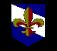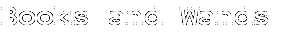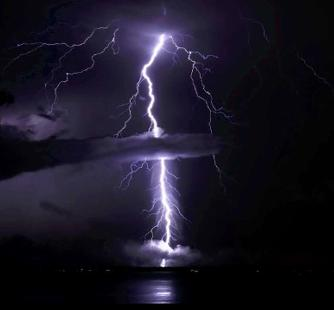The study of numbers and their possible meanings is referred to as numerology.  There are
many different techniques.  We used the method of digit summing.

It’s exactly what it sounds like. Generally, you’d take the date of birth and add each digit
separately, once you have your sum, if it’s a double digit, you’d add those two digits until you
get a single number.

Each number is assigned a meaning.  The following are the most common or the definitions
most widely accepted...generally. These are taken from wikipedia.
Number Meanings:
 Chinese 1 = Sure 2 = Easy 3 = Live 4 = Unlucky - pronunciation similar to 'death'. 5 = The self, or nothing/never 6 = Smooth path 7 = Together 8 = Sudden fortune/prosperity 9 = Long in time
So, for example:
 H
 E
 R
 M
 I
 O
 N
 E

 G
 R
 A
 N
 G
 E
 R
 8
 5
 18
 13
 9
 15
 14
 5

 7
 18
 1
 14
 7
 5
 18
 Hermione Granger
Hermione = 87 = 8+7 = 15 = 1+5 = 6 = Reaction(Smooth path)
Granger = 70 = 7 = Thought (Together)

To be thorough, you can add in the middle name:
 J
 A
 N
 E
 10
 1
 14
 5
Jane = 30 = 3 = Communication (Live)

In total: 6+7+3 = 16 = 7 = Thought (Together)
 Chinese: Lucky and Unlucky Numbers
 H
 A
 R
 R
 Y

 J
 A
 M
 E
 S

 P
 O
 T
 T
 E
 R
 8
 1
 18
 18
 25

 10
 1
 13
 5
 19

 16
 15
 20
 20
 5
 18For Nerds. By Nerds.
 ArithmancyA
1
 B
 2
C
3
D
4
E
5
F
6
G
7
H
8
I
9
J
10
K
11
L
12
M
13
 N 14 O 15 P 16 Q 17 R 18 S 19 T 20 U 21 V 22 W 23 X 24 Y 25 Z 26
 Arithmancy: Hermione
Hermione Jane Granger
Hermione: 6
Jane:         3
Granger:   7

Full Name Digit Sum: 7 = Thought (Together)
Birth Year Digit Sum: 9 = Completion (Long in Time)
 Arithmancy: Harry
Harry James Potter
Harry: 70 = 7 Thought (Together)
James: 48 = 12 = 3 Communication (Live)
Potter: 94 = 13 = 4 Creation (the unlucky number)

Full Name Digit Sum: 7 + 3 + 4=  14 = 5 Action (the Self)
Birth Year Digit Sum: 2 = Balance (Easy)
 Arithmancy: Ron
Ronald Bilius Weasley
Ronald: 64 = 10 = 1 Individual (Sure)
Bilius: 72 = 9 Completion (Long in Time)
Weasley: 90 = 9 Completion (Long in Time)

Full Name Digit Sum: 71+9+9 = 19 = 10 = 1 Individual (Sure)
Birth Year Digit Sum: 4 Creation (the unlucky number)
 1 March 19801+3+1980 = 1984 = 22 = 4Creation (the unlucky number)
 R
 O
 N
 A
 L
 D

 B
 I
 L
 I
 U
 S

 W
 E
 A
 S
 L
 E
 Y
 18
 15
 14
 1
 12
 4

 2
 9
 12
 9
 21
 19

 23
 5
 1
 19
 12
 5
 25
Date of Birth:
 19 September 197919 + 9 + 1979 = 2007 = 99 = Completion (Long in Time)
Date of Birth:
 31 July 198031+7+1980 = 2018 = 11 = 22 = Balance (Easy)
Date of Birth:
 Arithmancy: Luna
Luna Lovegood
Luna: 48 = 12 = 3 Communication (Live)
Lovegood: 95 = 15 = 5 Action (the Self)

Full Name Digit Sum: 3 + 5 = 8 Power (Sudden Fortune)
Birth Year Digit Sum: 1 Individual (Sure)
Date of Birth:
 We don’t know Luna’s birth date, but she was born in 1981.1+9+8+1 = 19 = 10 = 1Individual (Sure)
 L
 U
 N
 A

 L
 O
 V
 E
 G
 O
 O
 D
 12
 21
 14
 1

 12
 15
 22
 5
 7
 15
 15
 4
 Arithmancy: Neville
Neville Longbottom
Neville: 79 = 16 = 7 Thought (Together)
Longbottom: 133 = 7 Thought (Together)

Full Name Digit Sum: 7 + 7 = 14 = 5 Action (the Self)
Birth Year Digit Sum: 1 Individual (Sure)
Date of Birth:
 30 July 198030+7+1980 = 2017 = 10 = 1Individual (Sure)
 N
 E
 V
 I
 L
 L
 E

 L
 O
 N
 G
 B
 O
 T
 T
 O
 M
 14
 5
 22
 9
 12
 12
 5

 12
 15
 14
 7
 2
 15
 20
 20
 15
 13
 Arithmancy: Ginny
Date of Birth:
 11 August 198111+8+1981 = 2000 = 2Balance (Easy)
 G
 I
 N
 E
 V
 R
 A

 M
 O
 L
 L
 Y

 W
 E
 A
 S
 L
 E
 Y
 7
 9
 14
 5
 22
 18
 1

 13
 15
 12
 12
 25

 23
 5
 1
 19
 12
 5
 25Lucky
6 –     Flowing, Smooth; “everything goes smoothly”.  Considered one of the luckiest
numbers.
8 –     Fortune or Prosperity
9 –     Longlasting, enduring, long-term

Unlucky
4 –     It’s pronunciation in Chinese, Korean, and Japanese sounds similar to the words for
death in each
language.  It is considered the unluckiest numbers.
14 –   In Cantonese, the word is “sap sei,” which sounds like “sat sei.”  Sat sei translates
into “certainly die.”

The trepidation towards, and subsequent aversion of, the number 14 in Eastern cultures is very
similar to the Western idea of 13 being a bad sign.  The fear of the number 14 is called
Tetraphobia.

The fear is so strong in that part of the world that 4 and all other numbers containing it are
avoided, especially in the presence of sick or dying people.

The 4th, 14th, 24th, etc. floors are usually skipped in hotels, hospitals, offices.  In restaurants, or
at social gathering such as weddings, the 4th table is passed over.
 "Lightning Over New York City" photography by Christopher Imperato.All book series, movies, and images are owned by their authors, creators, publishers & production companies.No copyright infringement intended of any and all source material.No profits were made from this site.
Harry Potter
Arithmancy
Divination
Character Arcs
Muggle Studies
Under the Influence
Other Projects
Hunger Games
Book Notes
Movie Review
The Hobbit
Book Notes
Movie Review
Percy Jackson
Book Notes
Movie Review
Twilight
Book Notes
Movie Stills
Narnia
Book Notes
Movie Review
 Western 1 = Individual. Aggressor. Yang. 2 = Balance. Union. Yin. 3 = Communication/interaction. Neutrality. 4 = Creation. 5 = Action. 6 = Reaction/flux. Responsibility. 7 = Thought/consciousness. 8 = Power/Sacrifice. 9 = Completion.
For the Chinese, a number like 89 would be a very lucky number as it translates to something
like “you’ll have a lasting fortune” or “you’ll prosper for a long time”.

So let’s use, for example, Hermione.  Hermione’s birthday is 19 September 1979.

19+9+1979 = 2007 = 2+7 = 9 = Completion (Long in time)

There is also a method of designating numbers to each letter of the alphabet, then can you digit
sum from those values.